Sreenath S — Published On November 5, 2020 and Last Modified On October 26th, 2021

This article was published as a part of the Data Science Blogathon.

## Introduction

Many times we have come across this statement – Lasso regression causes sparsity while Ridge regression doesn’t! But I’m pretty sure that most of us might not have understood how exactly this works. Let’s try to understand this using calculus.

## Sparsity and Regularization

First, let’s understand what sparsity is. We are all familiar with the over-fitting problem, where the model performs extremely well on the observed data, while it fails to perform well on unseen data. We are also aware that lasso and ridge regressions are employed to solve this problem. The difference between the two approaches lies mainly in the way these algorithms perform regularization.

Regularization basically aims at proper feature selection to avoid over-fitting. Proper feature selection is achieved by optimizing the importance given to the features. Lasso regression achieves regularization by completely diminishing the importance given to some features (making the weight zero), whereas ridge regression achieves regularization by reducing the importance given to some of the features and not by nullifying the importance of the features. Thus, one can say that lasso regression causes sparsity while ridge regression doesn’t. But how does this actually happen?

## Unfolding the math

Let’s consider a regression scenario where ‘y’ is the predicted vector and ‘x’ is the feature matrix. Basically in any regression problem, we try to minimize the squared error. Let ‘β’ be the vector of parameters (weights of importance of features) and ‘p’ be the number of features.

Ridge regression is also called L2 regression as it uses the L2 norm for regularization. In ridge regression, we are trying to minimize the below function w.r.t ‘β’ in order to find the best ‘β’. Accordingly, we are trying to minimize the below function: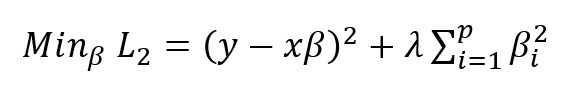The first term in the above expression is the squared error and the second term is the regularization. We are trying to understand whether minimizing L2 w.r.t β leads to sparsity (βi→0, for any i). Sparsity leads to feature selection as the weights of some features get diminished. Sparsity is achieved for a feature ‘i’ if the corresponding weight βi becomes zero. Here ‘λ’ is the regularization parameter. For simplicity, let p=1 and βi = β. Now,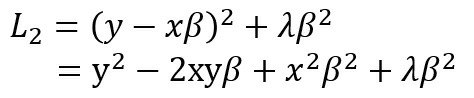Applying the first-order condition for local minima, we know that for ‘β’ to be a minima (β*),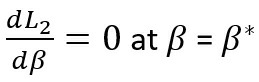or,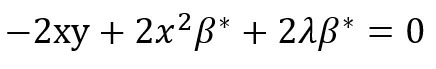which means,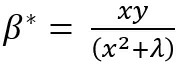For sparsity, β* = 0, This can happen only when λ→∝. So, it is clear that ridge regression doesn’t cause sparsity. It can cause sparsity only if the regularization parameter is infinity. So, in all practical cases, there will always be some weight associated with each feature, if we are employing ridge regression to achieve regularization.

Now, let’s discuss the case of lasso regression, which is also called L1 regression since it uses the L1 norm for regularization. In lasso regression, we try to solve the below minimization problem: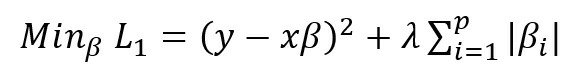For simplicity, let p=1 and βi = β. Now,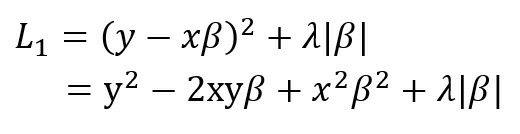Because of the term λ|β| it is clear that the function L1 is not continuous and hence not differentiable at the point of discontinuity. Hence the calculus approach which we followed in the case of ridge regression cannot be employed here to find the minima. But in the case of a discontinuous function, optimization theory states that optima occur at the point of discontinuities. It is possible that discontinuity occurs at β=0 and if this happens that leads to sparsity. To understand this better, let us visualize the above function.

From the above plot, it can be seen that as we increase the value of regularization parameter λ from 0.5 to 5, the function becomes less smooth and the point of discontinuity is at β=0, which is the minimum. This was the simplest case of regression with just a single feature and here lasso regression made that single feature sparse. So, it is clear that for a feature, it is possible for its corresponding weight β to become zero in lasso regression.

For ridge regression, the analysis was complete using calculus itself and we could prove that it is impossible for any of the weights to become zero. When we try to visualize the function L2 , this becomes even more clear. This function is smooth, without any discontinuities and hence it is differentiable throughout. From the plots, one may notice that the minimum occurs somewhere close to zero, but it is never at zero. As we keep increasing the value of λ from 0.5 to 5, the minima become closer to zero, though it never becomes zero!

## Example

Suppose we are building a linear model out of two features, we’ll have two coefficients (β1 and β2). For ridge regression, the penalty term, in this case, would be-

L2p = β12 + β22.

The linear regression model actually wants to maximize the values of β1 and β2, but also wants to minimize the penalty. The best possible way to minimize penalty to reduce the magnitude of the maximum of β1 or β2, as the penalty function is quadratic. Hence larger of the two coefficients will be subjected to shrinkage.

For better understanding let β1 = 10 and β2 = 1000. The regularization would shrink β2 more and β1  would almost remain the same since  β2 has been already made close to zero. Further shrinking β1 wouldn’t cause many effects on the whole function. Let’s say, β1 is shrunk to 8 and β2 to 100. This would shrink the overall penalty function from 1000100 to 10064, which is a significant change.

However, if we consider lasso regression, the L1 penalty would look like,

L1p = |β1| + |β2|

Shrinking β1 to 8 and β2 to 100 would minimize the penalty to 108 from 1010, which means in this case the change is not so significant just by shrinking the larger quantity. So, in the case of the L1 penalty, both the coefficients have to be shrunk to extremely small values, in order to achieve regularization. And in this whole process, some coefficients may shrink to zero.

## End Points

Here, I just tried to explain the sparsity exhibition in lasso and ridge regression using basic calculus and some visualizations. This was analyzed with the simple case of a single feature, just to get a sense of the function. The same kind of analysis is applicable when we have ‘p’ features. Imagine the visualization of the function in the p+1 dimensional space! In 3 dimensions (p=2), the lasso regression function would look like a diamond, and the ridge regression function would look like a sphere. Now, try visualizing for p+1 dimensions, and then you will get the answer to the question of sparsity in lasso and ridge regression.

I think we all understand the concept of regularization, but the intuitions and the math behind it are like a black-box for all of us. I hope this article helped in explaining the intuitions well.